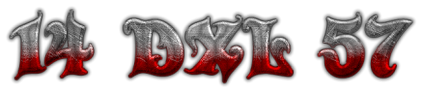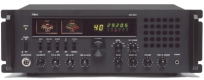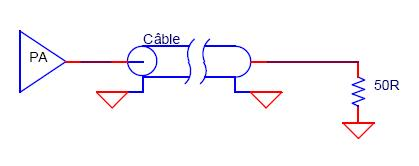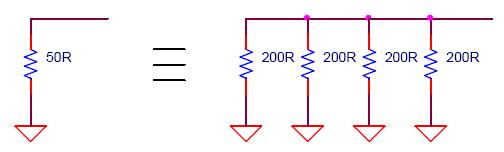Presentation :
It is often very interesting to have a load adapted to 50 Ohm.
Indeed it allows to be able to adapt an output of PA, or to make power measurements etc.Several parameters are to be studied for a correct load:
- dissipated power
- value and ROS
- etc
Dissipated power:
If your amplifier delivers an RF power of 5Watt for example,
care must be taken that the 50 Ohm load can withstand this power.
For this purpose resistors are placed in parallel in this way:The paralleling N resistors (in our example) makes it possible to increase the permissible power of the load by a factor N but also divides the value of the resistances by N.
If each 200 Ohm resistor can dissipate ¼ Watt,
the 4 resistors in parallel will dissipate 1 Watt.
The value of the load will be 200/4 = 50 Ohm (what we are looking for).
For a power of 10 Watt for example, we can use 10 resistors of 1 Watt.